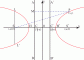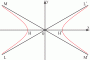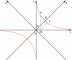I have forgotten

Coordinate

Definitions

Describes the abbreviations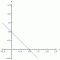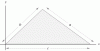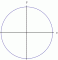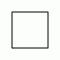Pairs of Straight Lines

An analysis of the equations associated with pairs of straight lines

Straight Line

Analysis of the Straight line and the Area of a Triangle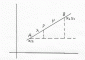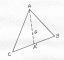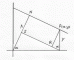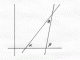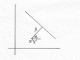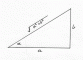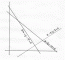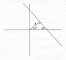Circle

An Analysis of a Circle and it's relationship with tangents and straight lines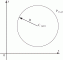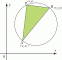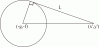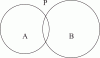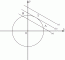Parabola

An analysis of the Parabola, its proprties, Chords and Tangents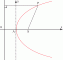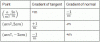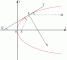Ellipse

Analysis of the Ellipse; Its Tangents and the Auxiliary Circle Chords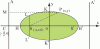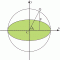Hyperbola

Analysis of the Hyperbola